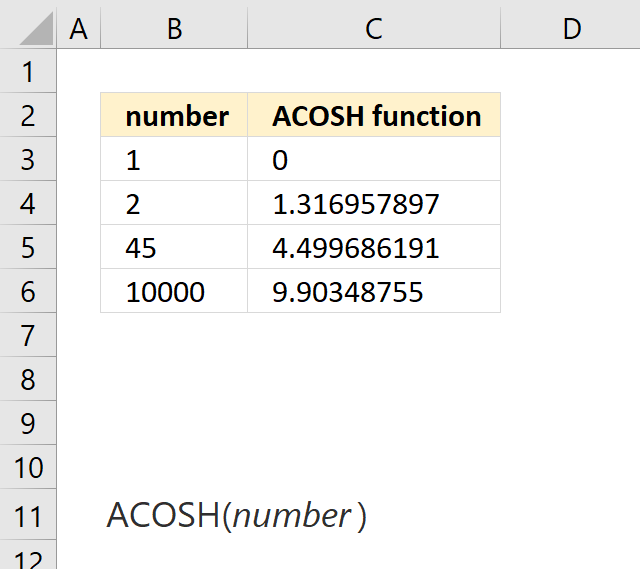Author: Oscar Cronquist Article last updated on March 27, 2018The ACOSH function calculates the inverse hyperbolic cosine of a number.

Formula in cell C3:

=ACOSH(B3)

ACOSH(number)

### Arguments

 number Required. Any real number equal to or greater than 1.

### Comments

ACOSH(COSH(number)) equals number.